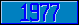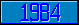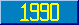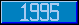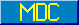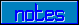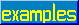## \$QUERY

### 7.1.5.15 \$Query

1. Let glvn be a variable reference of the form Name(s1, s2, ..., sq ) where sq may be the empty string. If glvn is unsubscripted, initialize V to the form Name(""); otherwise, initialize V to glvn.
2. If the last subscript of V is empty, Goto step e.
3. If \$D(V) \ 10 = 1, append the subscript "" to V, i.e., V is Name(s1, s2, ..., sq, "").
4. If V has no subscripts, return "".
5. Let s = \$O(V).
6. If s = "", truncate the last subscript off V, Goto step d.
7. If s '= "", replace the last subscript in V with s.
8. If \$D(V) # 2 = 1, return V formatted as a namevalue.
9. Goto step c.

If the value of \$Query(glvn) is not the empty string and glvn includes an environment, then the namevalue shall include the environment; otherwise the namevalue shall not include an environment.

If the argument of \$Query is a gvn, the naked indicator will become undefined and the value of \$Reference will become equal to the empty string.